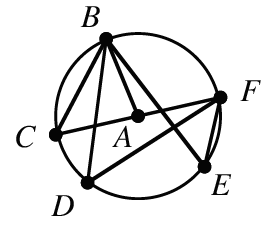### Home > INT2 > Chapter 10 > Lesson 10.2.2 > Problem10-66

10-66.

In $⊙A$ at right, $m∠C = 64°$. What is:

1. $m∠D$

How do the end points of $∠C$ and $∠D$ relate?

1. $m\overarc { B F }$

Notice $ΔCBA$. If A is the center, then what is true about the lines $AC$ and $AB$, and consequently $∠C$ and $∠B$?

What is true about the sum of $m∠CAB$ and $m∠BAF$?

$128°$

1. $m∠E$

See hint from part (a).

1. $m\overarc { C B F }$

If A is the center, then the line $CF$ must be the circle's diameter.

What does that mean for the the arc or semi-circle?

$180°$

1. $m∠BAF$

See hints for part (b).

1. $m∠BAC$

Use the answer from part (e) and hints from part (b).

$52°$